• 北京市
• 北京市
• 广东省
• 广东省
• 广州市
• 深圳市
• 珠海市
• 揭阳市
• 佛山市
• 肇庆市
• 潮州市
• 汕头市
• 阳江市
• 湛江市
• 韶关市
• 梅州市
• 河源市
• 惠州市
• 汕尾市
• 东莞市
• 中山市
• 云浮市
• 江门市
• 茂名市
• 清远市
• 辽宁省
• 辽宁省
• 沈阳市
• 大连市
• 鞍山市
• 抚顺市
• 本溪市
• 丹东市
• 锦州市
• 葫芦岛市
• 营口市
• 盘锦市
• 阜新市
• 辽阳市
• 铁岭市
• 朝阳市
• 吉林省
• 吉林省
• 长春市
• 吉林市
• 四平市
• 辽源市
• 通化市
• 白山市
• 松原市
• 白城市
• 延边朝鲜族自治州
• 黑龙江省
• 黑龙江省
• 哈尔滨市
• 齐齐哈尔市
• 鹤岗市
• 双鸭山市
• 鸡西市
• 大庆市
• 伊春市
• 牡丹江市
• 佳木斯市
• 七台河市
• 黑河市
• 绥化市
• 大兴安岭地区
• 上海市
• 上海市
• 黄浦区
• 卢湾区
• 徐汇区
• 长宁区
• 静安区
• 普陀区
• 闸北区
• 虹口区
• 杨浦区
• 闵行区
• 宝山区
• 嘉定区
• 浦东新区
• 金山区
• 松江区
• 青浦区
• 奉贤区
• 崇明县
• 江苏省
• 江苏省
• 南京市
• 徐州市
• 连云港市
• 淮安市
• 宿迁市
• 盐城市
• 扬州市
• 泰州市
• 南通市
• 镇江市
• 常州市
• 无锡市
• 苏州市
• 浙江省
• 浙江省
• 杭州市
• 宁波市
• 温州市
• 嘉兴市
• 湖州市
• 绍兴市
• 金华市
• 衢州市
• 舟山市
• 台州市
• 丽水市
• 安徽省
• 安徽省
• 合肥市
• 芜湖市
• 蚌埠市
• 淮南市
• 马鞍山市
• 淮北市
• 铜陵市
• 安庆市
• 黄山市
• 阜阳市
• 宿州市
• 巢湖市
• 六安市
• 亳州市
• 池州市
• 宣城市
• 滁州市
• 福建省
• 福建省
• 福州市
• 厦门市
• 三明市
• 莆田市
• 泉州市
• 漳州市
• 南平市
• 龙岩市
• 宁德市
• 江西省
• 江西省
• 南昌市
• 景德镇市
• 萍乡市
• 九江市
• 新余市
• 鹰潭市
• 赣州市
• 吉安市
• 宜春市
• 抚州市
• 上饶市
• 山东省
• 山东省
• 济南市
• 青岛市
• 淄博市
• 枣庄市
• 东营市
• 潍坊市
• 烟台市
• 威海市
• 济宁市
• 泰安市
• 日照市
• 莱芜市
• 临沂市
• 德州市
• 聊城市
• 滨州市
• 菏泽市
• 河南省
• 河南省
• 郑州市
• 开封市
• 洛阳市
• 平顶山市
• 焦作市
• 鹤壁市
• 新乡市
• 安阳市
• 濮阳市
• 许昌市
• 漯河市
• 三门峡市
• 南阳市
• 商丘市
• 信阳市
• 周口市
• 驻马店市
• 省直辖县级行政单位
• 湖北省
• 湖北省
• 武汉市
• 黄石市
• 襄樊市
• 十堰市
• 荆州市
• 宜昌市
• 荆门市
• 鄂州市
• 孝感市
• 黄冈市
• 咸宁市
• 随州市
• 恩施土家族苗族自治州
• 省直辖县级行政单位
• 湖南省
• 湖南省
• 长沙市
• 株洲市
• 湘潭市
• 衡阳市
• 邵阳市
• 岳阳市
• 常德市
• 张家界市
• 益阳市
• 郴州市
• 永州市
• 怀化市
• 娄底市
• 湘西土家族苗族自治州
• 广西壮族自治区
• 广西壮族自治区
• 南宁市
• 柳州市
• 桂林市
• 梧州市
• 北海市
• 防城港市
• 钦州市
• 贵港市
• 玉林市
• 百色市
• 贺州市
• 河池市
• 来宾市
• 崇左市
• 海南省
• 海南省
• 海口市
• 三亚市
• 省直辖行政单位
• 重庆市
• 重庆市
• 渝中区
• 大渡口区
• 江北区
• 沙坪坝区
• 九龙坡区
• 南岸区
• 北碚区
• 万盛区
• 双桥区
• 渝北区
• 巴南区
• 万州区
• 涪陵区
• 黔江区
• 长寿区
• 合川市
• 永川市
• 江津市
• 南川市
• 綦江县
• 潼南县
• 铜梁县
• 大足县
• 荣昌县
• 璧山县
• 垫江县
• 武隆县
• 丰都县
• 城口县
• 梁平县
• 开县
• 巫溪县
• 巫山县
• 奉节县
• 云阳县
• 忠县
• 石柱土家族自治县
• 彭水苗族土家族自治县
• 酉阳土家族苗族自治县
• 秀山土家族苗族自治县
• 四川省
• 四川省
• 成都市
• 自贡市
• 攀枝花市
• 泸州市
• 德阳市
• 绵阳市
• 广元市
• 遂宁市
• 内江市
• 乐山市
• 南充市
• 宜宾市
• 广安市
• 达州市
• 眉山市
• 雅安市
• 巴中市
• 资阳市
• 阿坝藏族羌族自治州
• 甘孜藏族自治州
• 凉山彝族自治州
• 贵州省
• 贵州省
• 贵阳市
• 六盘水市
• 遵义市
• 安顺市
• 铜仁地区
• 毕节地区
• 黔西南布依族苗族自治州
• 黔东南苗族侗族自治州
• 黔南布依族苗族自治州
• 云南省
• 云南省
• 昆明市
• 曲靖市
• 玉溪市
• 保山市
• 昭通市
• 思茅地区
• 临沧地区
• 丽江地区
• 文山壮族苗族自治州
• 红河哈尼族彝族自治州
• 西双版纳傣族自治州
• 楚雄彝族自治州
• 大理白族自治州
• 德宏傣族景颇族自治州
• 怒江傈僳族自治州
• 迪庆藏族自治州
• 西藏自治区
• 西藏自治区
• 拉萨市
• 那曲地区
• 昌都地区
• 山南地区
• 日喀则地区
• 阿里地区
• 林芝地区
• 陕西省
• 陕西省
• 西安市
• 铜川市
• 宝鸡市
• 咸阳市
• 渭南市
• 延安市
• 汉中市
• 榆林市
• 安康市
• 商洛市
• 甘肃省
• 甘肃省
• 兰州市
• 金昌市
• 白银市
• 天水市
• 嘉峪关市
• 武威市
• 张掖市
• 平凉市
• 酒泉市
• 庆阳市
• 定西地区
• 甘南藏族自治州
• 临夏回族自治州
• 陇南地区
• 青海省
• 青海省
• 西宁市
• 海东地区
• 海北藏族自治州
• 黄南藏族自治州
• 海南藏族自治州
• 果洛藏族自治州
• 玉树藏族自治州
• 海西蒙古族藏族自治州
• 宁夏回族自治区
• 宁夏回族自治区
• 银川市
• 石嘴山市
• 吴忠市
• 固原市
• 新疆维吾尔自治区
• 新疆维吾尔自治区
• 乌鲁木齐市
• 克拉玛依市
• 自治区直辖县级行政单位
• 吐鲁番地区
• 哈密地区
• 和田地区
• 阿克苏地区
• 喀什地区
• 克孜勒苏柯尔克孜自治州
• 昌吉回族自治州
• 博尔塔拉蒙古自治州
• 伊犁哈萨克自治州
• 塔城地区
• 阿勒泰地区
• 巴音郭楞蒙古自治州

• 补益安神
• 补益安神
• 耳鼻喉用药
• 耳鼻喉用药
• 肝胆胰用药
• 肝胆胰用药
• 胆结石
• 胆囊炎
• 肝癌
• 肝炎
• 肝硬化
• 黄疸
• 两肋胀痛
• 其它
• 其它肝病用药
• 胰腺癌
• 胰腺炎
• 脂肪肝
• 转氨酶增高
• 呼吸系统类
• 呼吸系统类
• 肺癌
• 肺结核
• 咳嗽咳痰
• 咯血
• 其它
• 上呼吸道感染
• 哮喘
• 胸腔积液
• 咽痛音哑
• 抗寄生虫类
• 抗寄生虫类
• 阿米巴病
• 滴虫病
• 蛔虫病
• 螨虫感染
• 蛲虫病
• 疟疾
• 其它
• 丝虫病
• 解热镇痛类
• 解热镇痛类
• 癌症疼痛
• 创伤性疼痛(手术-外伤-烧伤)
• 发热头痛
• 风湿疼痛
• 感冒-病毒性感冒
• 肌肉关节痛
• 其他类
• 祛暑用药
• 痛经
• 牙痛
• 抗过敏用药
• 抗过敏用药
• 过敏性鼻炎
• 其它
• 过敏性荨麻疹
• 过敏性哮喘
• 抗结核及麻风类
• 抗结核及麻风类
• 结核病
• 麻风病
• 抗菌消炎类
• 抗菌消炎类
• 扁桃体炎
• 带状疱疹
• 单纯疱疹
• 胆囊炎
• 肺炎
• 风疹
• 甲癣
• 尖锐湿疣
• 阑尾炎
• 痢疾
• 流感
• 霉菌性阴道炎
• 尿道炎
• 其它
• 气管炎
• 肾盂肾炎
• 水痘
• 体癣
• 胃炎
• 咽喉炎
• 胰腺炎
• 抗肿瘤药
• 抗肿瘤药
• 抗代谢
• 抗生素类
• 抗肿瘤辅助药
• 天然抗肿瘤药
• 烷化剂
• 泌尿系统类
• 泌尿系统类
• 尿毒症
• 尿路结石
• 尿频尿急尿痛
• 其它
• 肾癌
• 肾病综合征
• 肾炎
• 水肿
• 腰膝酸软
• 免疫功能调节
• 免疫功能调节
• 干燥综合症
• 红斑狼疮
• 类风湿
• 免疫功能低下
• 其它
• 器官移植
• 内分泌及代谢类
• 内分泌及代谢类
• 肥胖症
• 激素药物
• 甲状腺功能减退症
• 甲状腺功能亢进
• 尿崩症
• 其它
• 糖尿病
• 痛风
• 皮肤用药
• 皮肤用药
• 其它类
• 其它类
• 清热解毒
• 清热解毒
• 神经系统类
• 神经系统类
• 痴呆
• 癫痫
• 多发性神经炎
• 肌无力
• 精神分裂症
• 面瘫
• 脑动脉硬化
• 帕金森
• 偏头痛
• 其它
• 神经官能症
• 神经衰弱
• 失眠多梦
• 眩晕耳鸣
• 抑郁症
• 晕车晕船
• 水电解质及酸碱平衡
• 水电解质及酸碱平衡
• 低血钾
• 低血糖
• 其它水电解质紊乱
• 维生素及营养类
• 维生素及营养类
• 补钙补锌
• 补铁补硒
• 复合维生素
• 其它微量元素
• 叶酸类
• 营养药
• 胃肠疾病类
• 胃肠疾病类
• 便秘
• 便血
• 恶心呕吐
• 返酸打嗝
• 腹痛腹泻
• 结肠癌
• 呕血
• 其它
• 食道癌
• 食欲不振
• 胃癌
• 急慢性胃炎
• 消化不良
• 胃及十二指肠溃疡
• 痔疮
• 心脑血管类
• 心脑血管类
• 病毒性心肌炎
• 动脉硬化
• 肺动脉高压
• 高血压
• 高血脂
• 冠心病
• 其它
• 心功能不全
• 心慌心悸
• 心肌病
• 心绞痛
• 心力衰竭
• 心律失常
• 胸闷胸痛
• 中风偏瘫
• 血液疾病类
• 血液疾病类
• 白细胞减少
• 白血病
• 贫血
• 其它
• 血小板减少
• 止血药
• 眼科用药
• 眼科用药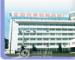• 哈药集团制药总厂
• [药企地址]哈尔滨市南岗区学府路109号
• [联系电话]0451-86662926,86666391
• [企业简介]

哈药集团制药总厂隶属于哈药集团有限公司，企业性质为中外合资股份制企业，是中国重点抗生素生产基?...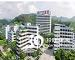• 华润三九医药股份有限公司
• [药企地址]深圳市罗湖区银湖路口（北环大道1028号）
• [联系电话]0755-83360999
• [企业简介]

华润三九医药股份有限公司(简称“华润三九”)是大型国有控股医药上市公司，前身为深圳南?...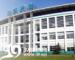• 北京双鹤药业股份有限公司
• [药企地址]北京市朝阳区望京利泽东二路1号
• [联系电话]010-64742227
• [企业简介]

双鹤研究院是北京双鹤药业股份有限公司所属的医药专业研发机构。双鹤研究院主要由双鹤药业的研发机?...• 江苏恒瑞医药股份有限公司
• [药企地址]连云港市经济技术开发区昆仑山路7号
• [联系电话]0518-85465745,85475710
• [企业简介]

江苏恒瑞医药股份有限公司始建于1970年，2000年在上海证券交易所上市，股票代码600276，是国内最大?...• 辉瑞制药有限公司
• [药企地址]大连市经济技术开发区大庆路22号
• [联系电话]0411-87615400
• [企业简介]

辉瑞公司为人类及动物的健康发现、开发、生产和推广各种领先的处方药以及许多世界最驰名的消费产品?...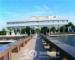• 上海罗氏制药有限公司
• [药企地址]上海浦东新区龙东大道1100号
• [联系电话]021-28922888
• [企业简介]

罗氏始创于1896年，总部位于瑞士巴塞尔，在制药和诊断领域是世界领先的以研发为基础的健康事业公司?...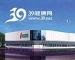• 江苏康缘美域生物医药有限公司
• [药企地址].连云港经济技术开发区江宁工业城,连云港市经济技术开发区泰山北路58号
• [联系电话]0518-85521999
• [企业简介]

江苏康缘药业股份有限公司，是国家新医药产业化基地的重点骨干企业、国家中药现代化示范企业、国家?...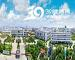• 浙江海正药业股份有限公司
• [药企地址]浙江省台州市椒江区外沙路
• [联系电话]0576-88827890,88088385,88089080,88087600
• [企业简介]

浙江海正药业股份有限公司始创于1956年，位于中国东南沿海的港口城市---浙江省台州市。目前海正已成...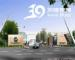• 北京紫竹药业有限公司
• [药企地址]北京朝阳区朝阳北路27号
• [联系电话]010-65483355
• [企业简介]

北京紫竹药业有限公司，是由具有三十多年历史的北京第三制药厂于2000年改制而成的一家大型综合性高?...• 上海新先锋药业有限公司
• [药企地址]上海浦东张江高科技园区张江路92号
• [联系电话]021-58552452
• [企业简介]

上海医药（集团）有限公司抗生素事业部的战略定位：以抗生素为主，做精、做强、做大非专利的处方药?...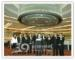• 珠海联邦制药股份有限公司中山分公司
• [药企地址]广东省中山市坦洲镇嘉联路12号
• [联系电话]0760) 86655816 0760) 86655310
• [企业简介]

联邦制药1990年成立于香港。1994年，投资数亿港元创建珠海联邦制药股份有限公司，以先进的制药技术?...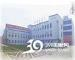• 石药集团中诺药业(石家庄)有限公司
• [药企地址]河北省石家庄市工农路188号
• [联系电话]0311-83803616,83805386,85666188
• [企业简介]

石药集团中诺药业概况介绍：

石药集团中诺药业（石家庄）有限公司成立于2002年...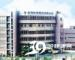• 深圳致君制药有限公司
• [药企地址]深圳市宝安区观澜高新技术产业园区
• [联系电话]0755-82433388
• [企业简介]

深圳致君制药有限公司作为国药控股旗下深圳一致药业的核心企业，其前身为深圳市制药厂，成立于1985?...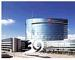• 葛兰素史克(天津)有限公司
• [药企地址]天津经济技术开发区第五大街65号
• [联系电话]022-23025008
• [企业简介]

葛兰素史克公司在中国的历史最早可追溯至20世纪初叶。自20世纪80年代以来，在中国政府改革开放政策?...• 香港澳美制药厂
• [药企地址]香港新界工业村宏富街8号
• [联系电话]00852-26750230
• [企业简介]

澳美制药是香港第一个现代化的、独立的、具有多功能生产厂房的制药企业,经过多年的发展，已经壮大成...

1 2 3 4 5 6 7 8 9 首页 上一页 下一页 末页